Скачать презентацию INTRODUCTION TO ARTIFICIAL INTELLIGENCE Massimo Poesio LECTURE 2

61692125892d4ca523bbeccaf2ece0d8.ppt

• Количество слайдов: 54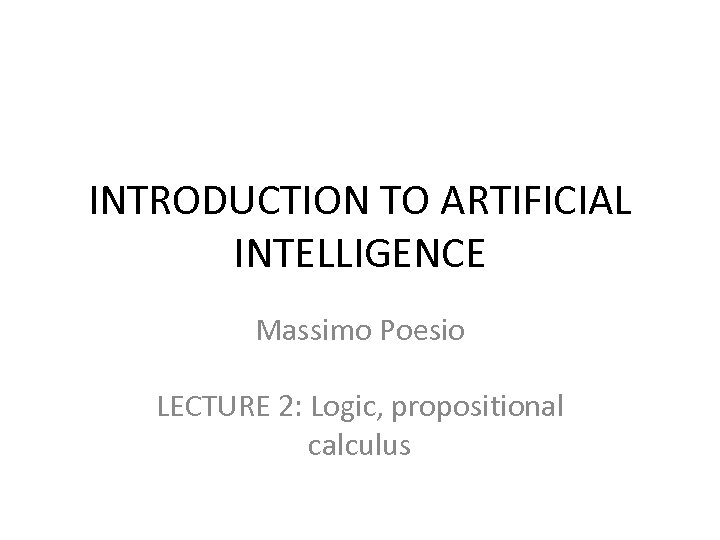INTRODUCTION TO ARTIFICIAL INTELLIGENCE Massimo Poesio LECTURE 2: Logic, propositional calculus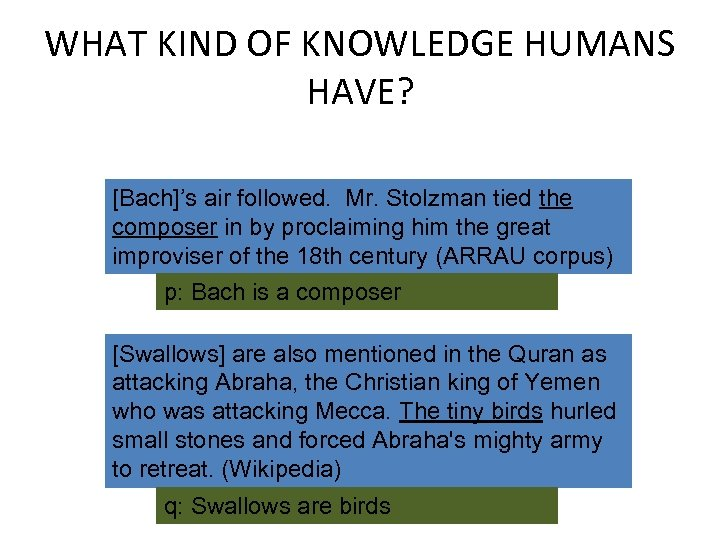WHAT KIND OF KNOWLEDGE HUMANS HAVE? [Bach]’s air followed. Mr. Stolzman tied the composer in by proclaiming him the great improviser of the 18 th century (ARRAU corpus) p: Bach is a composer [Swallows] are also mentioned in the Quran as attacking Abraha, the Christian king of Yemen who was attacking Mecca. The tiny birds hurled small stones and forced Abraha's mighty army to retreat. (Wikipedia) q: Swallows are birds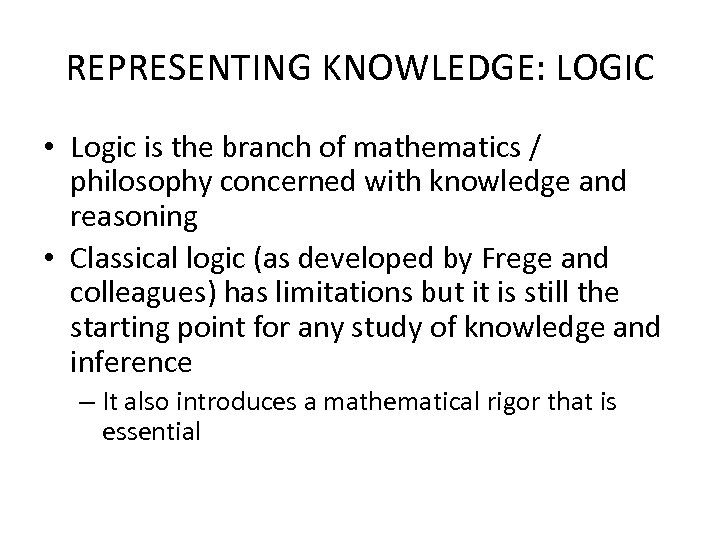REPRESENTING KNOWLEDGE: LOGIC • Logic is the branch of mathematics / philosophy concerned with knowledge and reasoning • Classical logic (as developed by Frege and colleagues) has limitations but it is still the starting point for any study of knowledge and inference – It also introduces a mathematical rigor that is essential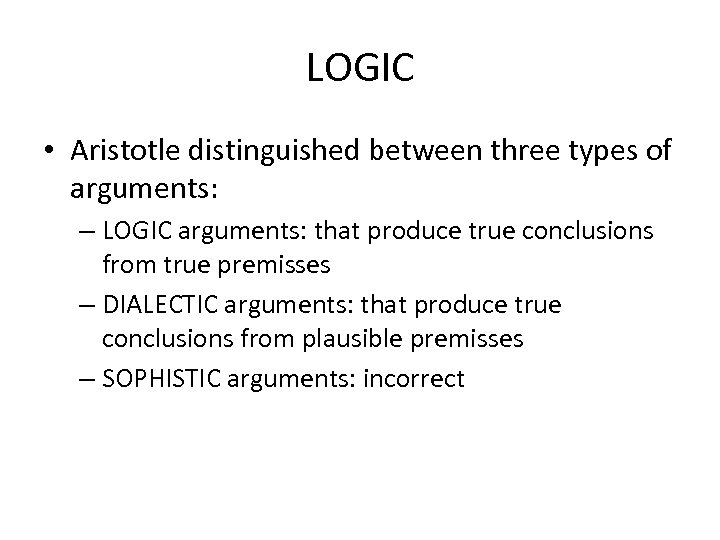LOGIC • Aristotle distinguished between three types of arguments: – LOGIC arguments: that produce true conclusions from true premisses – DIALECTIC arguments: that produce true conclusions from plausible premisses – SOPHISTIC arguments: incorrect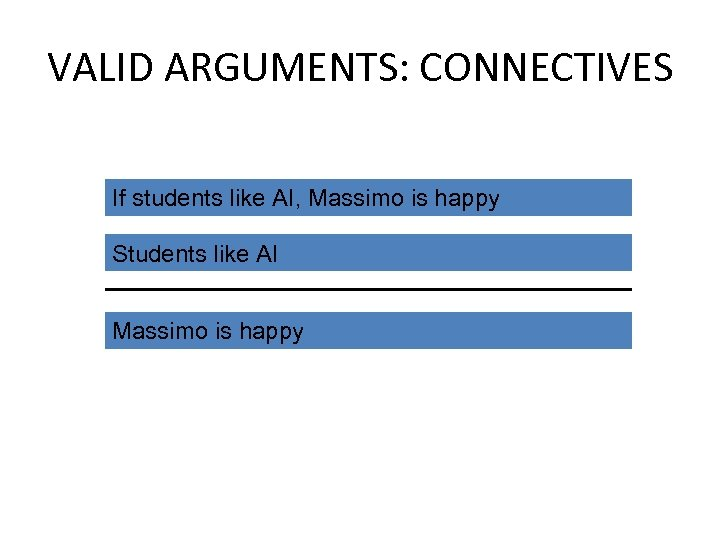VALID ARGUMENTS: CONNECTIVES If students like AI, Massimo is happy Students like AI Massimo is happy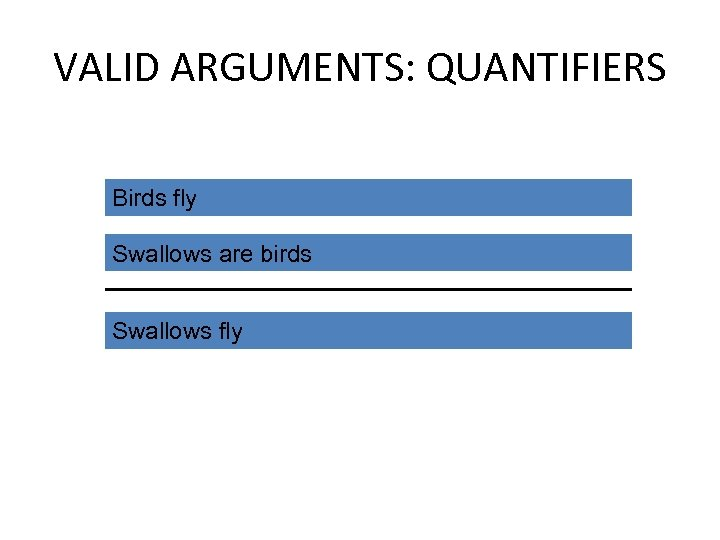VALID ARGUMENTS: QUANTIFIERS Birds fly Swallows are birds Swallows fly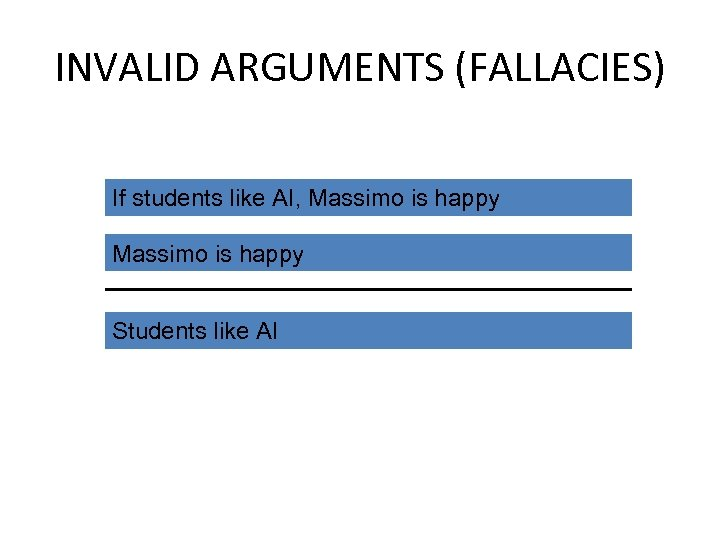INVALID ARGUMENTS (FALLACIES) If students like AI, Massimo is happy Students like AI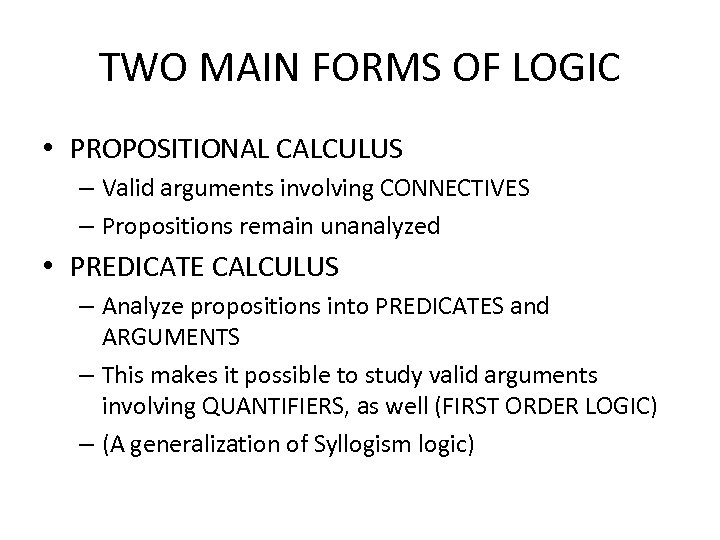TWO MAIN FORMS OF LOGIC • PROPOSITIONAL CALCULUS – Valid arguments involving CONNECTIVES – Propositions remain unanalyzed • PREDICATE CALCULUS – Analyze propositions into PREDICATES and ARGUMENTS – This makes it possible to study valid arguments involving QUANTIFIERS, as well (FIRST ORDER LOGIC) – (A generalization of Syllogism logic)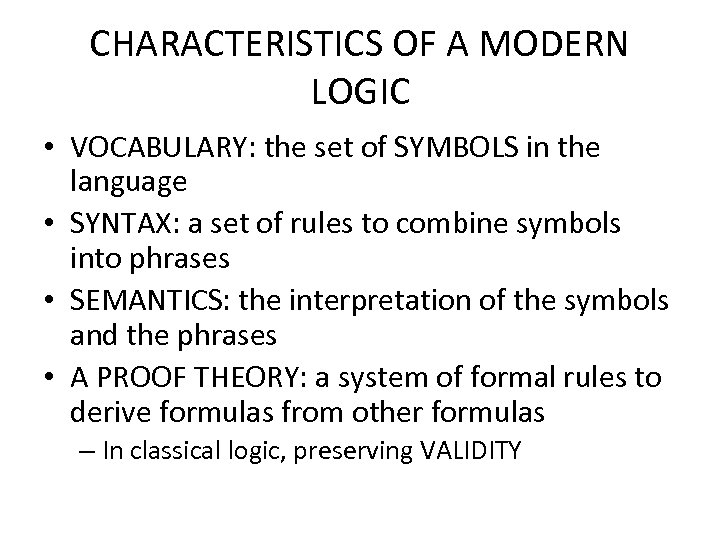CHARACTERISTICS OF A MODERN LOGIC • VOCABULARY: the set of SYMBOLS in the language • SYNTAX: a set of rules to combine symbols into phrases • SEMANTICS: the interpretation of the symbols and the phrases • A PROOF THEORY: a system of formal rules to derive formulas from other formulas – In classical logic, preserving VALIDITY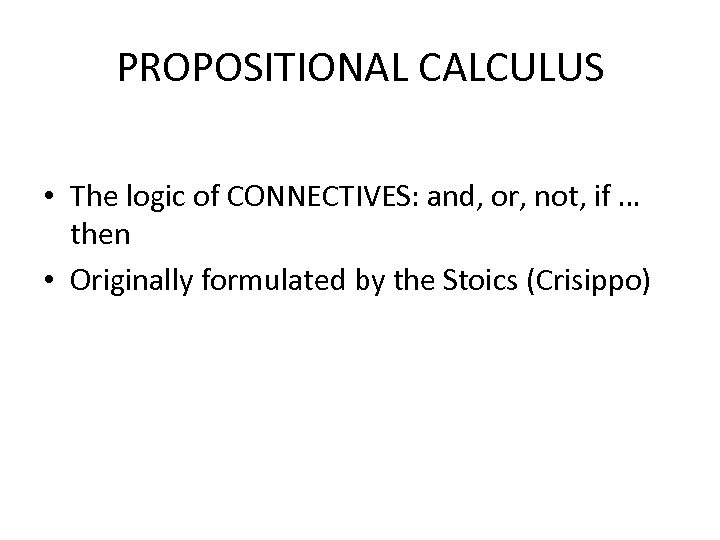PROPOSITIONAL CALCULUS • The logic of CONNECTIVES: and, or, not, if … then • Originally formulated by the Stoics (Crisippo)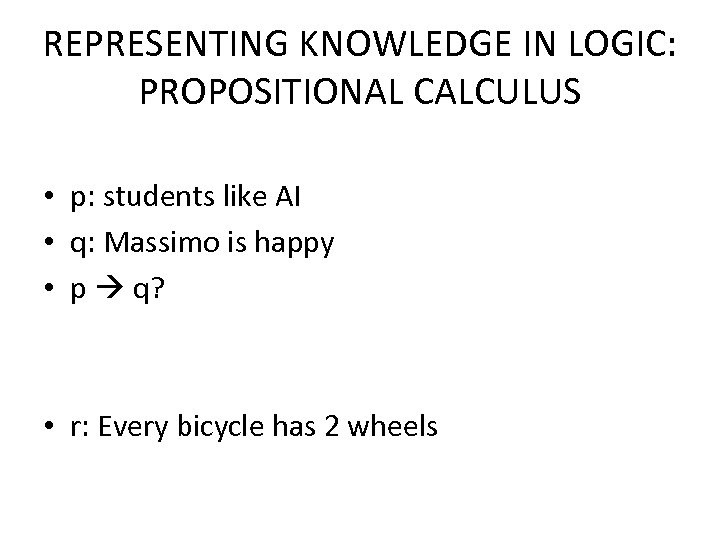REPRESENTING KNOWLEDGE IN LOGIC: PROPOSITIONAL CALCULUS • p: students like AI • q: Massimo is happy • p q? • r: Every bicycle has 2 wheels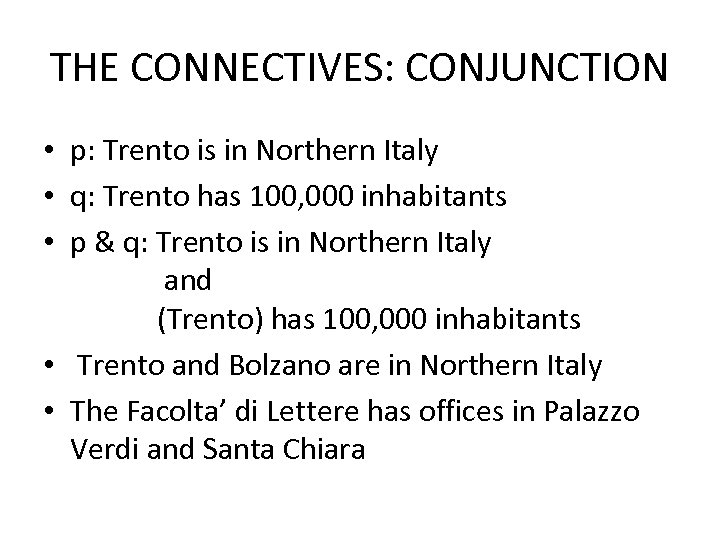THE CONNECTIVES: CONJUNCTION • p: Trento is in Northern Italy • q: Trento has 100, 000 inhabitants • p & q: Trento is in Northern Italy and (Trento) has 100, 000 inhabitants • Trento and Bolzano are in Northern Italy • The Facolta’ di Lettere has offices in Palazzo Verdi and Santa Chiara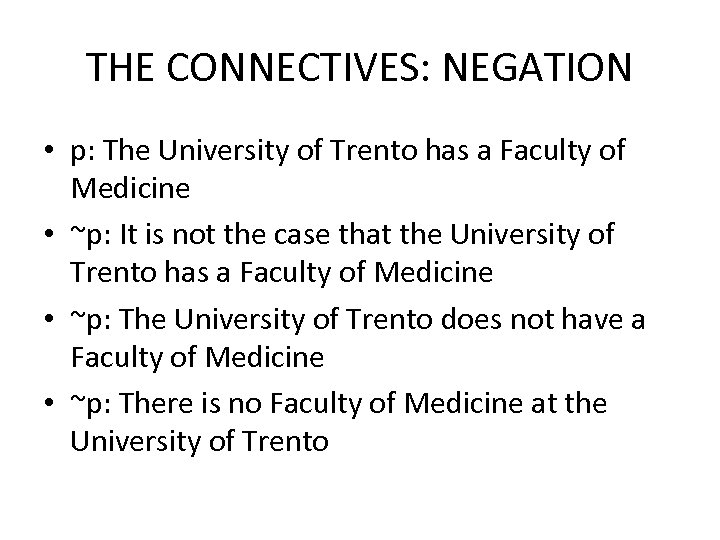THE CONNECTIVES: NEGATION • p: The University of Trento has a Faculty of Medicine • ~p: It is not the case that the University of Trento has a Faculty of Medicine • ~p: The University of Trento does not have a Faculty of Medicine • ~p: There is no Faculty of Medicine at the University of Trento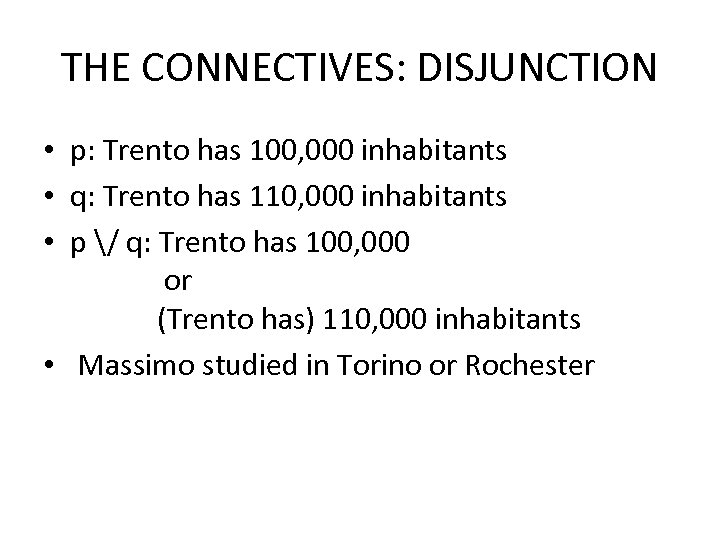THE CONNECTIVES: DISJUNCTION • p: Trento has 100, 000 inhabitants • q: Trento has 110, 000 inhabitants • p / q: Trento has 100, 000 or (Trento has) 110, 000 inhabitants • Massimo studied in Torino or Rochester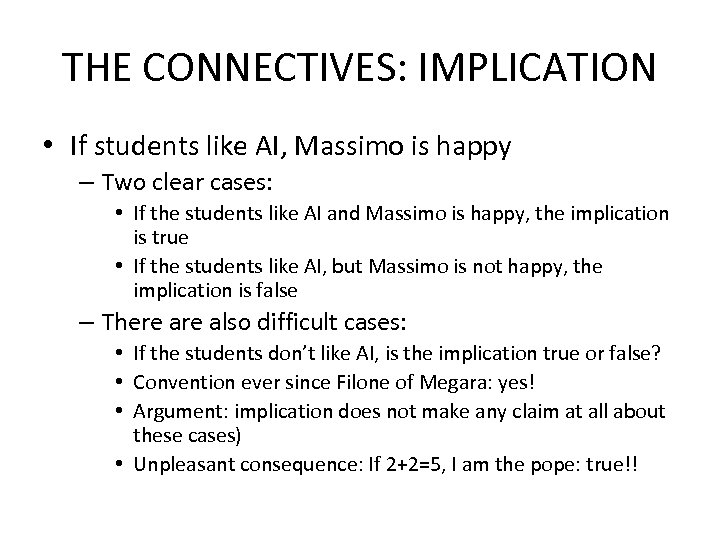THE CONNECTIVES: IMPLICATION • If students like AI, Massimo is happy – Two clear cases: • If the students like AI and Massimo is happy, the implication is true • If the students like AI, but Massimo is not happy, the implication is false – There also difficult cases: • If the students don’t like AI, is the implication true or false? • Convention ever since Filone of Megara: yes! • Argument: implication does not make any claim at all about these cases) • Unpleasant consequence: If 2+2=5, I am the pope: true!!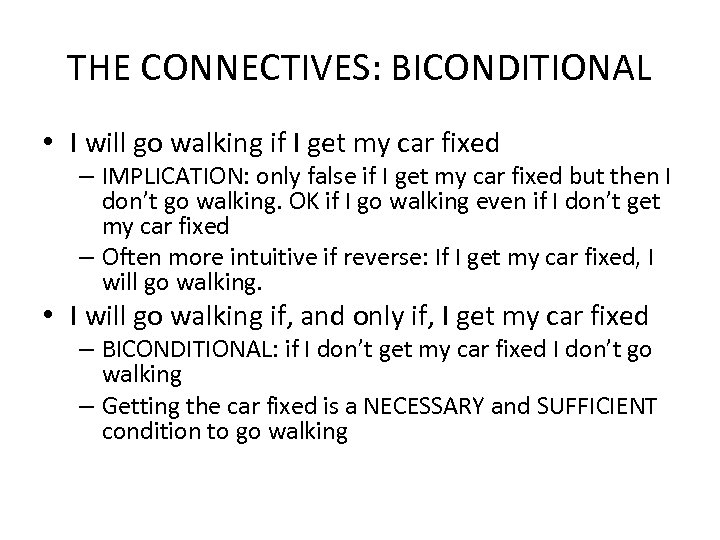THE CONNECTIVES: BICONDITIONAL • I will go walking if I get my car fixed – IMPLICATION: only false if I get my car fixed but then I don’t go walking. OK if I go walking even if I don’t get my car fixed – Often more intuitive if reverse: If I get my car fixed, I will go walking. • I will go walking if, and only if, I get my car fixed – BICONDITIONAL: if I don’t get my car fixed I don’t go walking – Getting the car fixed is a NECESSARY and SUFFICIENT condition to go walking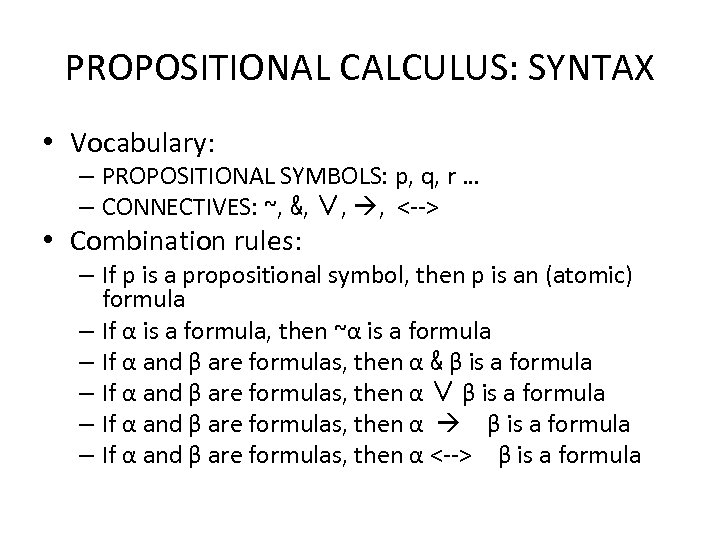PROPOSITIONAL CALCULUS: SYNTAX • Vocabulary: – PROPOSITIONAL SYMBOLS: p, q, r … – CONNECTIVES: ~, &, ∨, , <--> • Combination rules: – If p is a propositional symbol, then p is an (atomic) formula – If α is a formula, then ~α is a formula – If α and β are formulas, then α & β is a formula – If α and β are formulas, then α ∨ β is a formula – If α and β are formulas, then α <--> β is a formula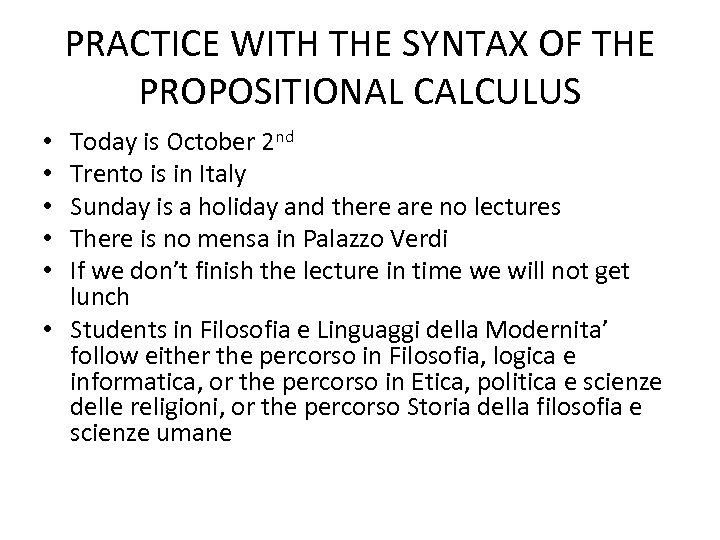PRACTICE WITH THE SYNTAX OF THE PROPOSITIONAL CALCULUS Today is October 2 nd Trento is in Italy Sunday is a holiday and there are no lectures There is no mensa in Palazzo Verdi If we don’t finish the lecture in time we will not get lunch • Students in Filosofia e Linguaggi della Modernita’ follow either the percorso in Filosofia, logica e informatica, or the percorso in Etica, politica e scienze delle religioni, or the percorso Storia della filosofia e scienze umane • • •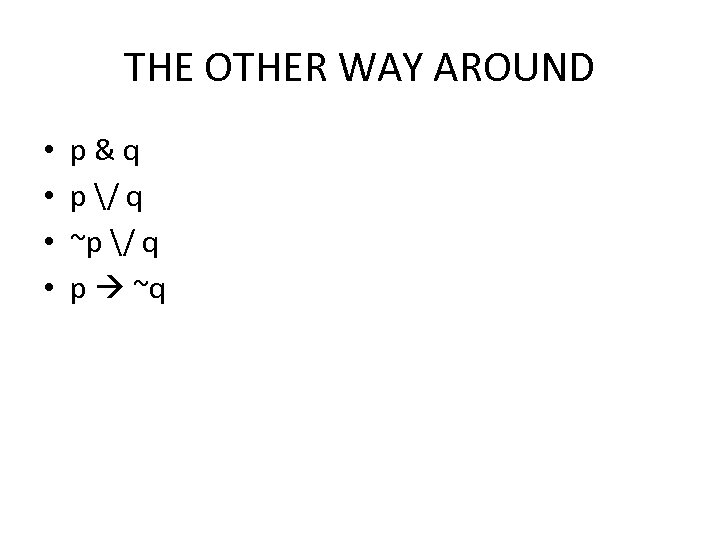THE OTHER WAY AROUND • • p&q p / q ~p / q p ~q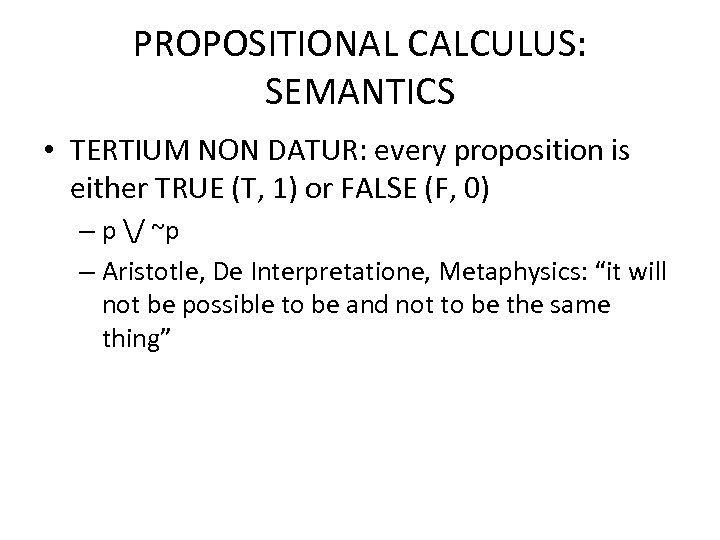PROPOSITIONAL CALCULUS: SEMANTICS • TERTIUM NON DATUR: every proposition is either TRUE (T, 1) or FALSE (F, 0) – p / ~p – Aristotle, De Interpretatione, Metaphysics: “it will not be possible to be and not to be the same thing”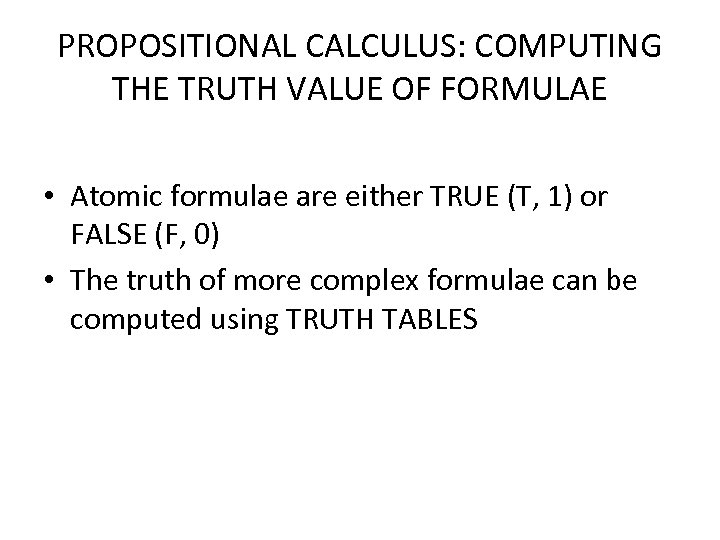PROPOSITIONAL CALCULUS: COMPUTING THE TRUTH VALUE OF FORMULAE • Atomic formulae are either TRUE (T, 1) or FALSE (F, 0) • The truth of more complex formulae can be computed using TRUTH TABLES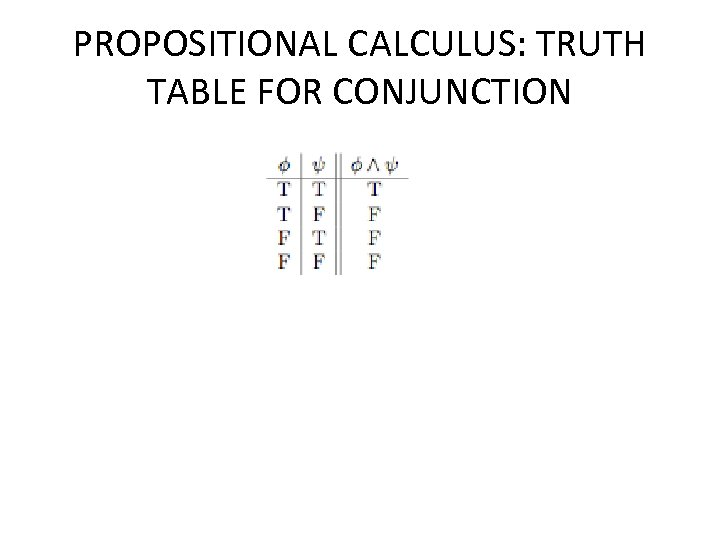PROPOSITIONAL CALCULUS: TRUTH TABLE FOR CONJUNCTION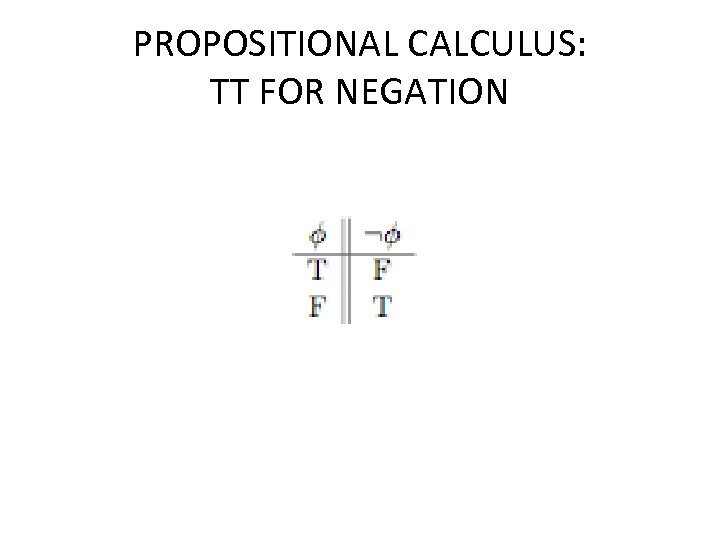PROPOSITIONAL CALCULUS: TT FOR NEGATION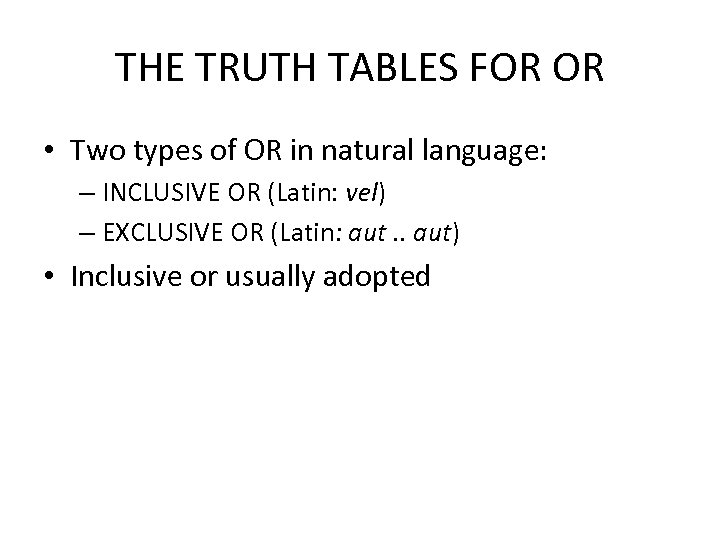THE TRUTH TABLES FOR OR • Two types of OR in natural language: – INCLUSIVE OR (Latin: vel) – EXCLUSIVE OR (Latin: aut. . aut) • Inclusive or usually adopted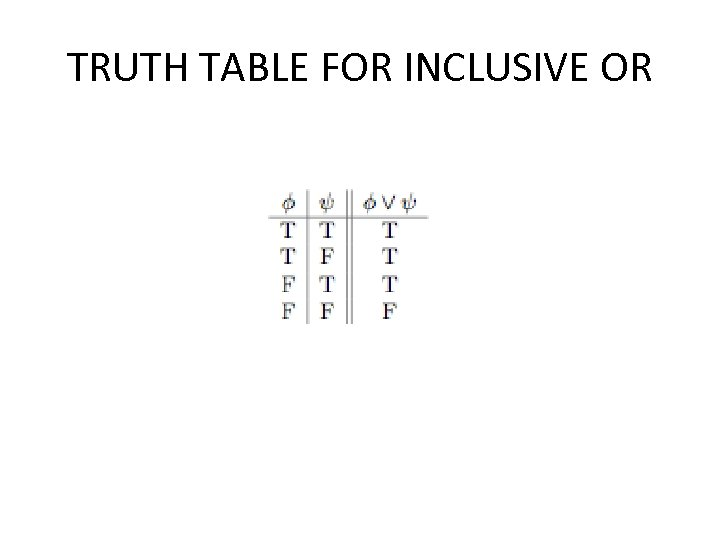TRUTH TABLE FOR INCLUSIVE OR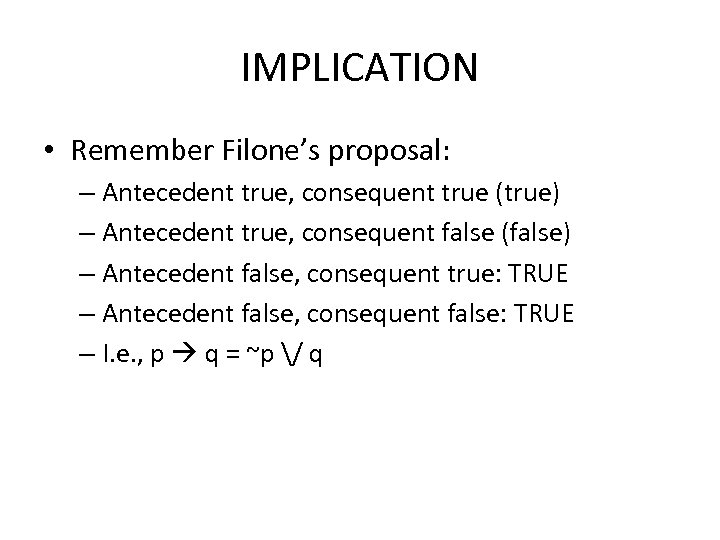IMPLICATION • Remember Filone’s proposal: – Antecedent true, consequent true (true) – Antecedent true, consequent false (false) – Antecedent false, consequent true: TRUE – Antecedent false, consequent false: TRUE – I. e. , p q = ~p / q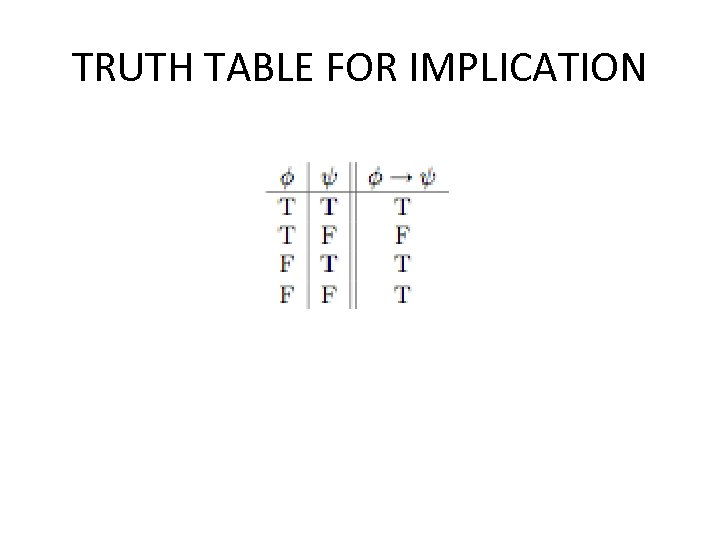TRUTH TABLE FOR IMPLICATION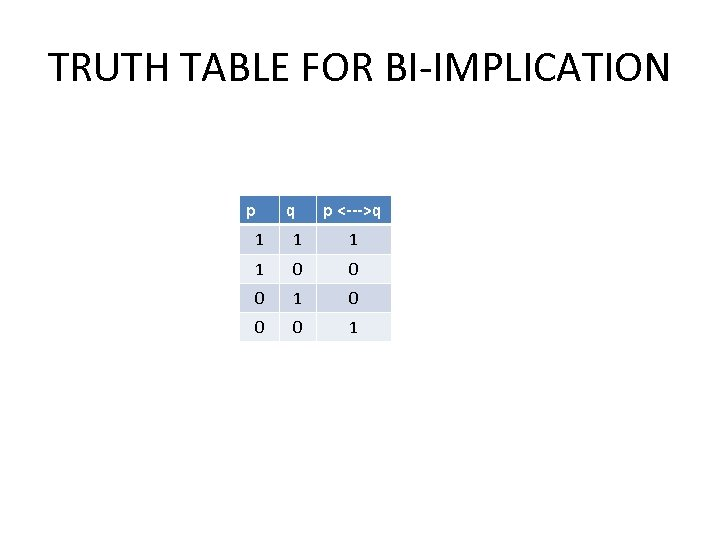TRUTH TABLE FOR BI-IMPLICATION p q p <--->q 1 1 0 0 0 1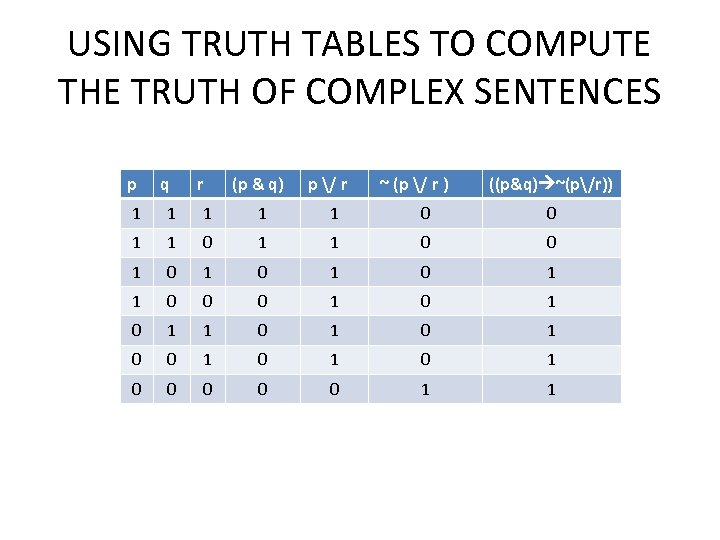USING TRUTH TABLES TO COMPUTE THE TRUTH OF COMPLEX SENTENCES p q r (p & q) p / r ~ (p / r ) ((p&q) ~(p/r)) 1 1 1 0 0 1 0 1 1 0 0 0 1 0 1 0 1 0 0 0 0 0 1 1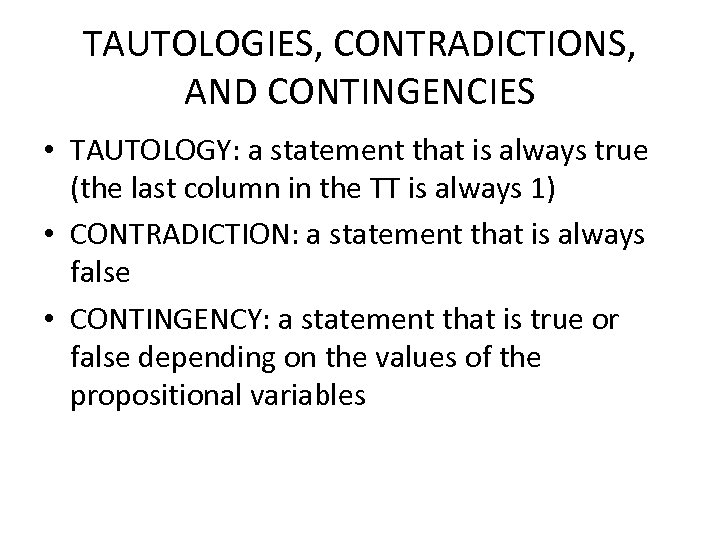TAUTOLOGIES, CONTRADICTIONS, AND CONTINGENCIES • TAUTOLOGY: a statement that is always true (the last column in the TT is always 1) • CONTRADICTION: a statement that is always false • CONTINGENCY: a statement that is true or false depending on the values of the propositional variables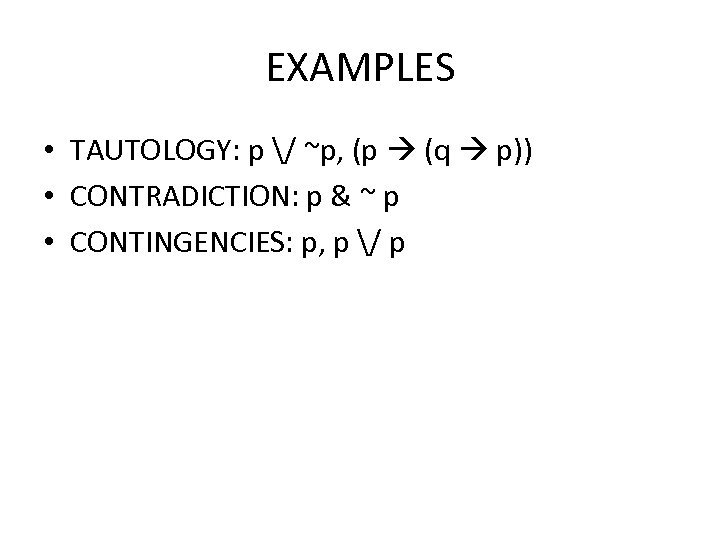EXAMPLES • TAUTOLOGY: p / ~p, (p (q p)) • CONTRADICTION: p & ~ p • CONTINGENCIES: p, p / p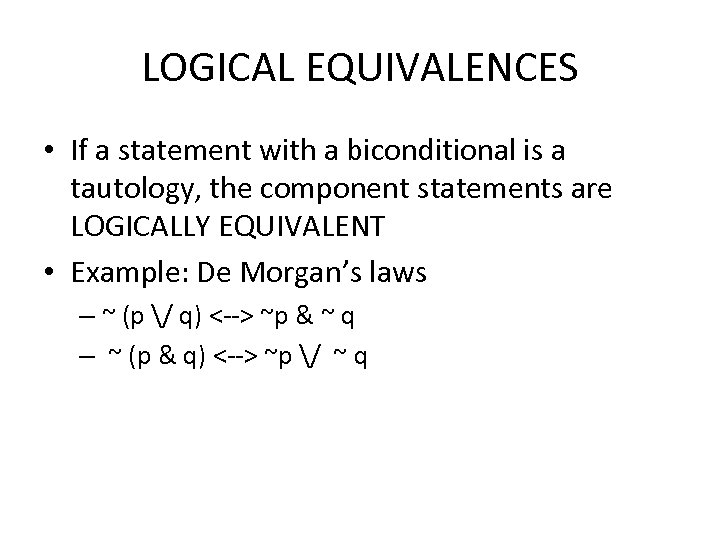LOGICAL EQUIVALENCES • If a statement with a biconditional is a tautology, the component statements are LOGICALLY EQUIVALENT • Example: De Morgan’s laws – ~ (p / q) <--> ~p & ~ q – ~ (p & q) <--> ~p / ~ q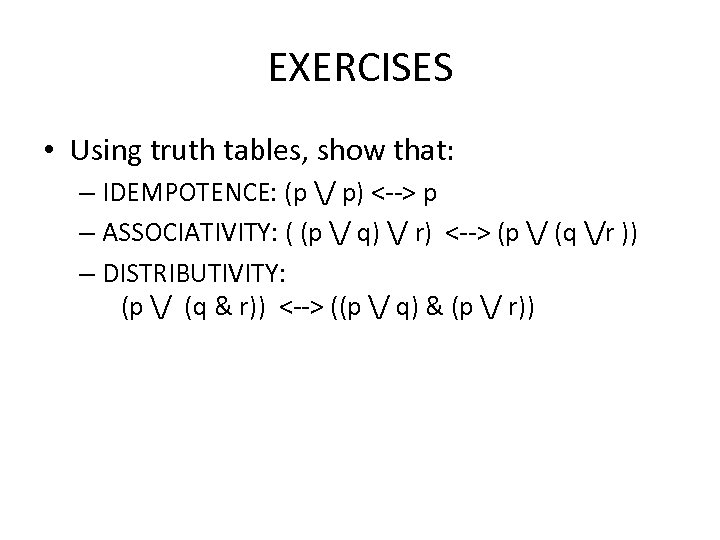EXERCISES • Using truth tables, show that: – IDEMPOTENCE: (p / p) <--> p – ASSOCIATIVITY: ( (p / q) / r) <--> (p / (q /r )) – DISTRIBUTIVITY: (p / (q & r)) <--> ((p / q) & (p / r))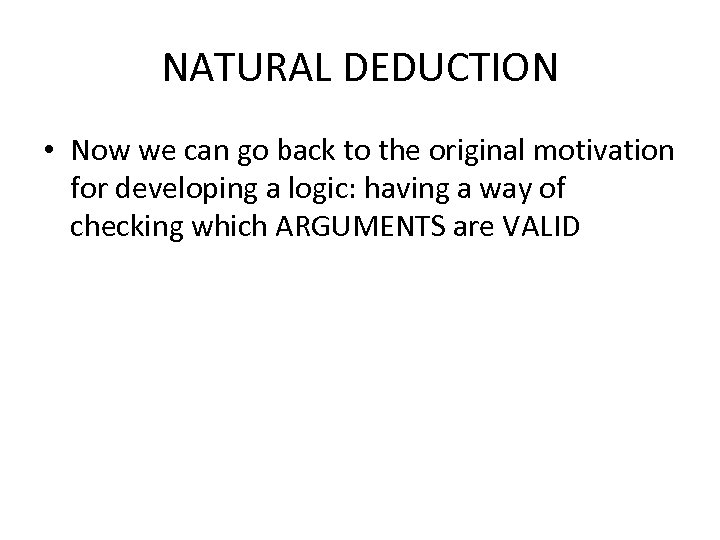NATURAL DEDUCTION • Now we can go back to the original motivation for developing a logic: having a way of checking which ARGUMENTS are VALID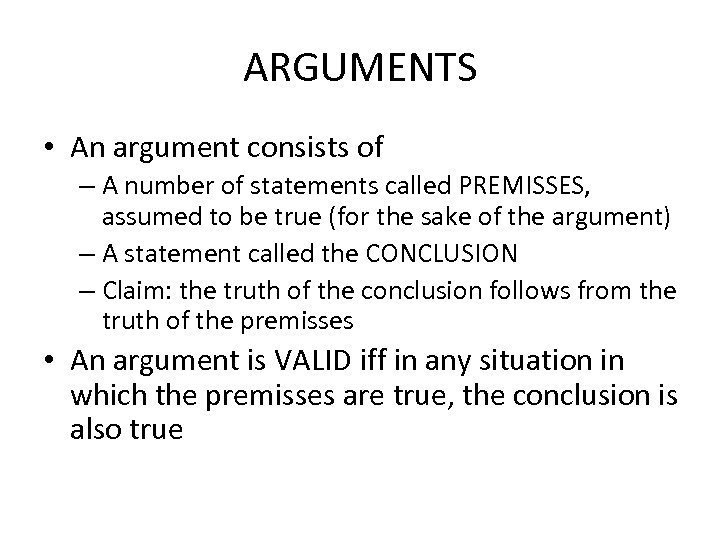ARGUMENTS • An argument consists of – A number of statements called PREMISSES, assumed to be true (for the sake of the argument) – A statement called the CONCLUSION – Claim: the truth of the conclusion follows from the truth of the premisses • An argument is VALID iff in any situation in which the premisses are true, the conclusion is also true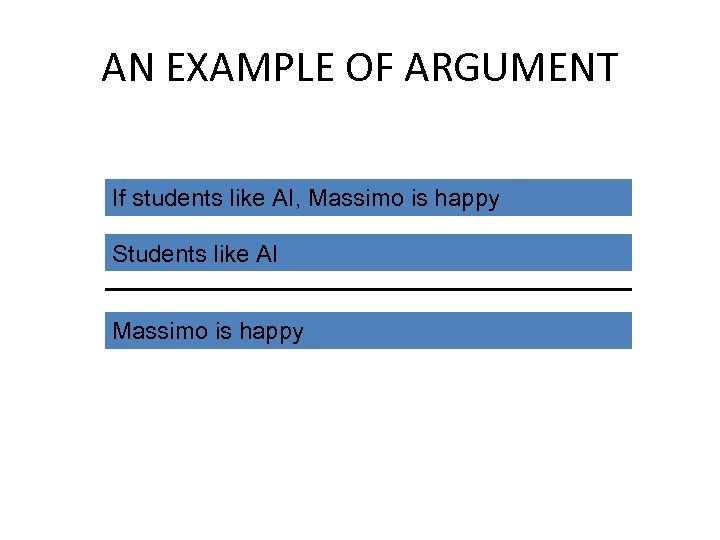AN EXAMPLE OF ARGUMENT If students like AI, Massimo is happy Students like AI Massimo is happy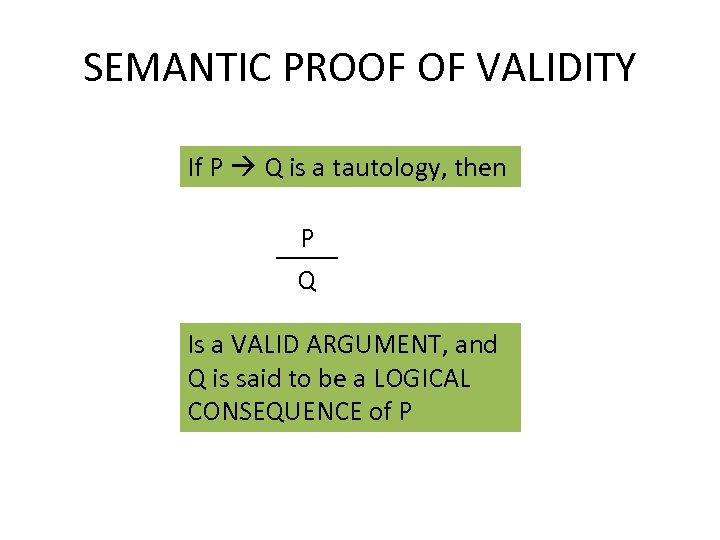SEMANTIC PROOF OF VALIDITY If P Q is a tautology, then P Q Is a VALID ARGUMENT, and Q is said to be a LOGICAL CONSEQUENCE of P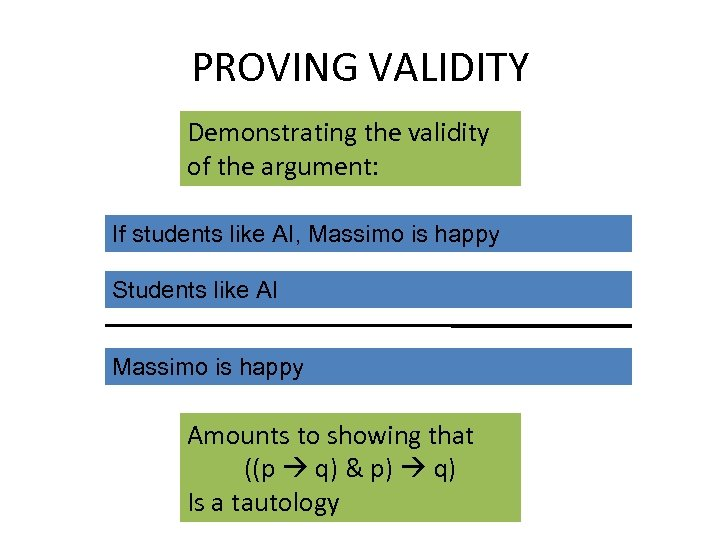PROVING VALIDITY Demonstrating the validity of the argument: If students like AI, Massimo is happy Students like AI Massimo is happy Amounts to showing that ((p q) & p) q) Is a tautology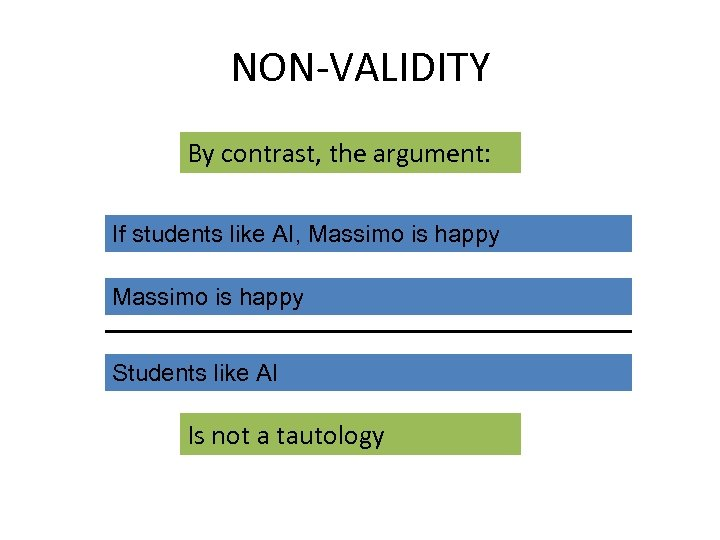NON-VALIDITY By contrast, the argument: If students like AI, Massimo is happy Students like AI Is not a tautology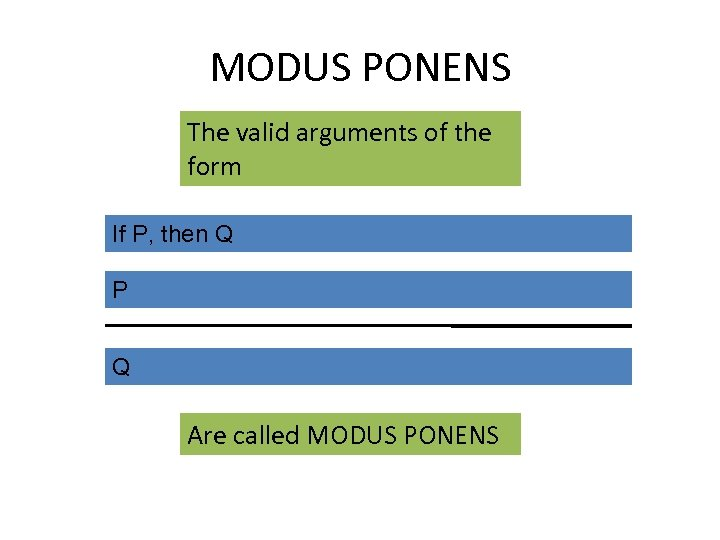MODUS PONENS The valid arguments of the form If P, then Q P Q Are called MODUS PONENS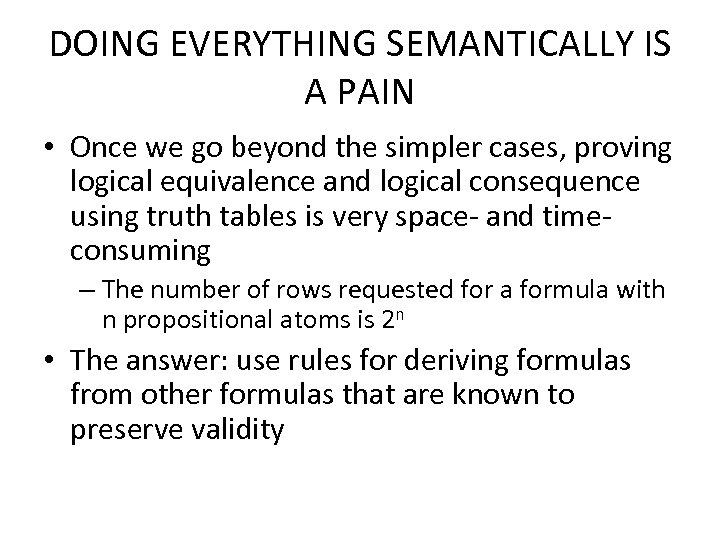DOING EVERYTHING SEMANTICALLY IS A PAIN • Once we go beyond the simpler cases, proving logical equivalence and logical consequence using truth tables is very space- and timeconsuming – The number of rows requested for a formula with n propositional atoms is 2 n • The answer: use rules for deriving formulas from other formulas that are known to preserve validity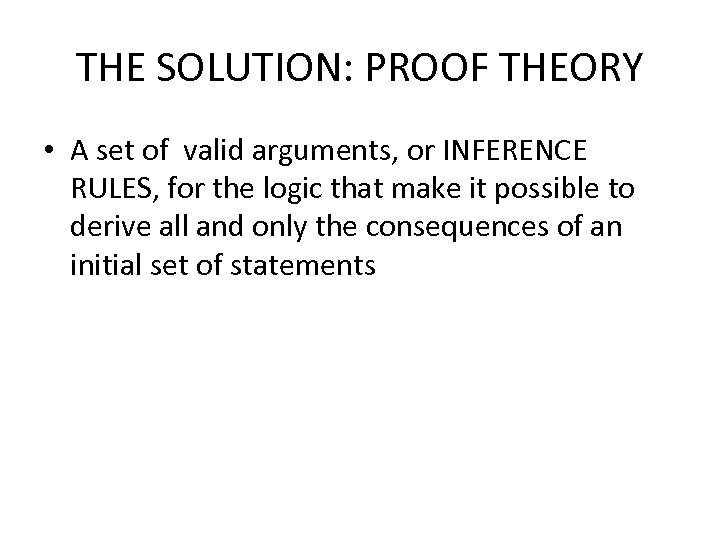THE SOLUTION: PROOF THEORY • A set of valid arguments, or INFERENCE RULES, for the logic that make it possible to derive all and only the consequences of an initial set of statements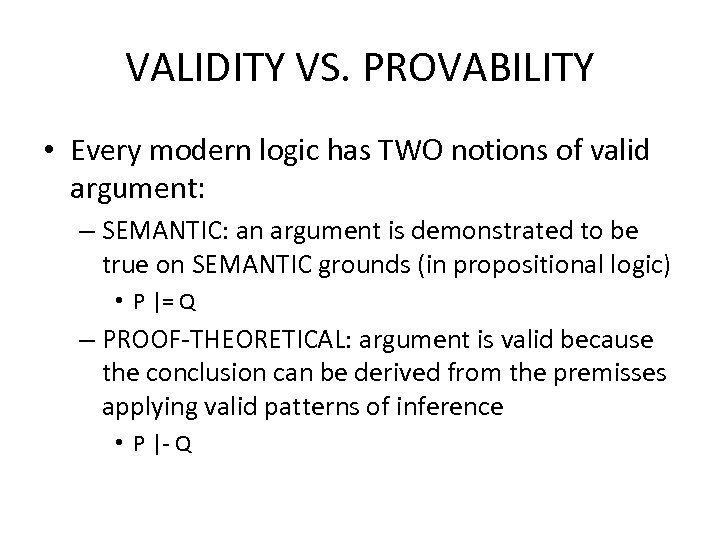VALIDITY VS. PROVABILITY • Every modern logic has TWO notions of valid argument: – SEMANTIC: an argument is demonstrated to be true on SEMANTIC grounds (in propositional logic) • P |= Q – PROOF-THEORETICAL: argument is valid because the conclusion can be derived from the premisses applying valid patterns of inference • P |- Q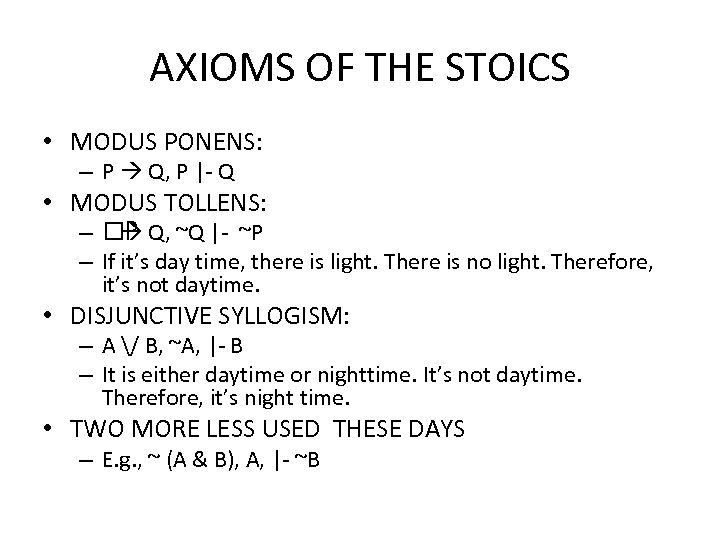AXIOMS OF THE STOICS • MODUS PONENS: – P Q, P |- Q • MODUS TOLLENS: – P Q, ~Q |- ~P – If it’s day time, there is light. There is no light. Therefore, it’s not daytime. • DISJUNCTIVE SYLLOGISM: – A / B, ~A, |- B – It is either daytime or nighttime. It’s not daytime. Therefore, it’s night time. • TWO MORE LESS USED THESE DAYS – E. g. , ~ (A & B), A, |- ~B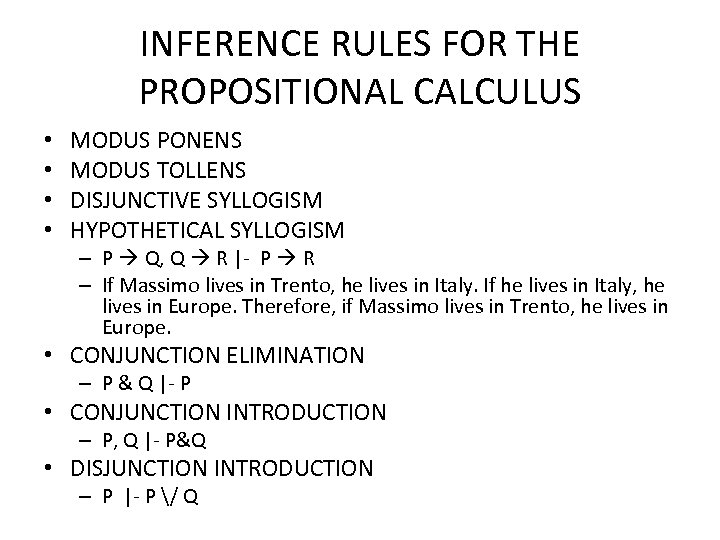INFERENCE RULES FOR THE PROPOSITIONAL CALCULUS • • MODUS PONENS MODUS TOLLENS DISJUNCTIVE SYLLOGISM HYPOTHETICAL SYLLOGISM – P Q, Q R |- P R – If Massimo lives in Trento, he lives in Italy. If he lives in Italy, he lives in Europe. Therefore, if Massimo lives in Trento, he lives in Europe. • CONJUNCTION ELIMINATION – P & Q |- P • CONJUNCTION INTRODUCTION – P, Q |- P&Q • DISJUNCTION INTRODUCTION – P |- P / Q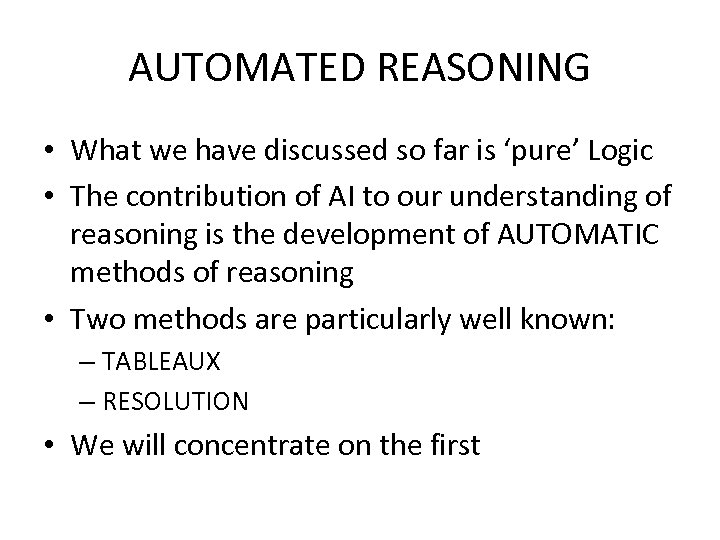AUTOMATED REASONING • What we have discussed so far is ‘pure’ Logic • The contribution of AI to our understanding of reasoning is the development of AUTOMATIC methods of reasoning • Two methods are particularly well known: – TABLEAUX – RESOLUTION • We will concentrate on the first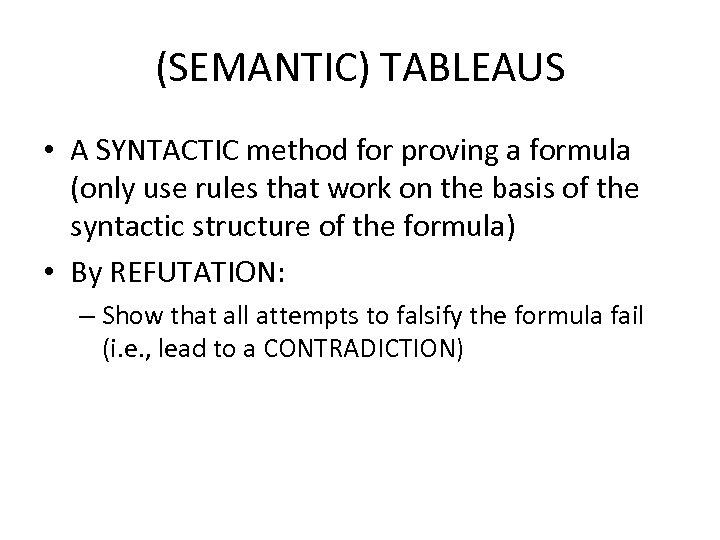(SEMANTIC) TABLEAUS • A SYNTACTIC method for proving a formula (only use rules that work on the basis of the syntactic structure of the formula) • By REFUTATION: – Show that all attempts to falsify the formula fail (i. e. , lead to a CONTRADICTION)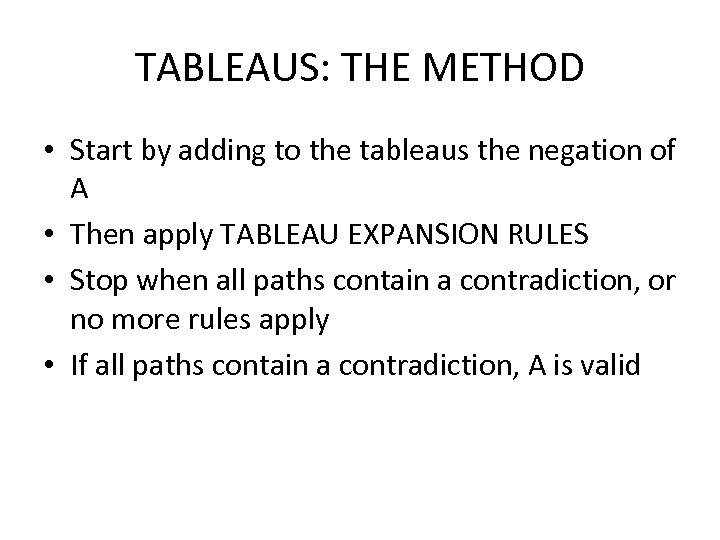TABLEAUS: THE METHOD • Start by adding to the tableaus the negation of A • Then apply TABLEAU EXPANSION RULES • Stop when all paths contain a contradiction, or no more rules apply • If all paths contain a contradiction, A is valid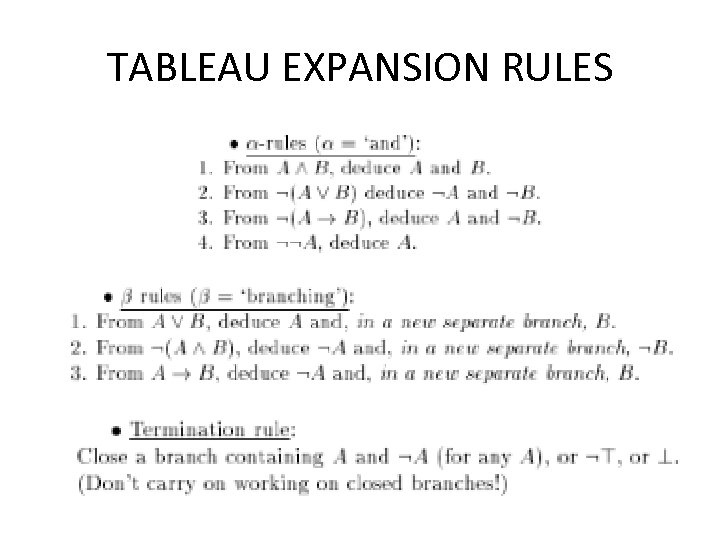TABLEAU EXPANSION RULES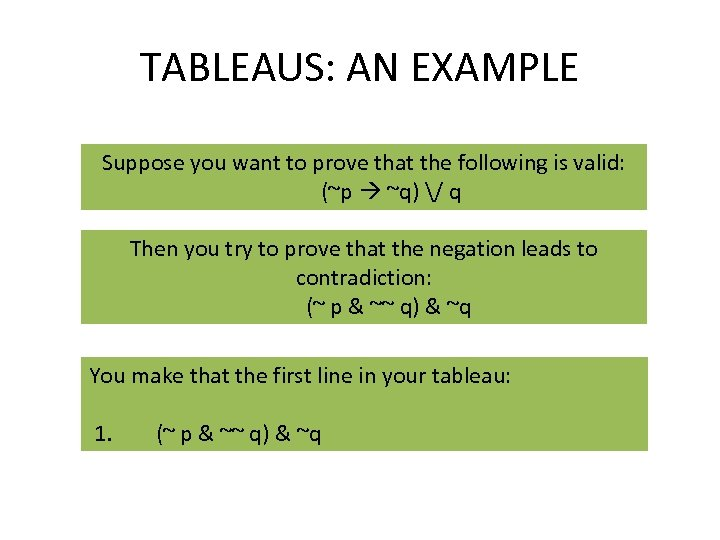TABLEAUS: AN EXAMPLE Suppose you want to prove that the following is valid: (~p ~q) / q Then you try to prove that the negation leads to contradiction: (~ p & ~~ q) & ~q You make that the first line in your tableau: 1. (~ p & ~~ q) & ~q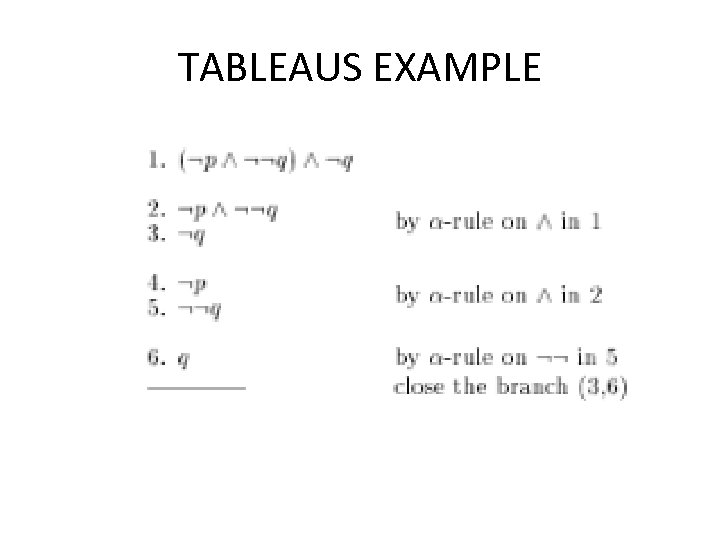TABLEAUS EXAMPLE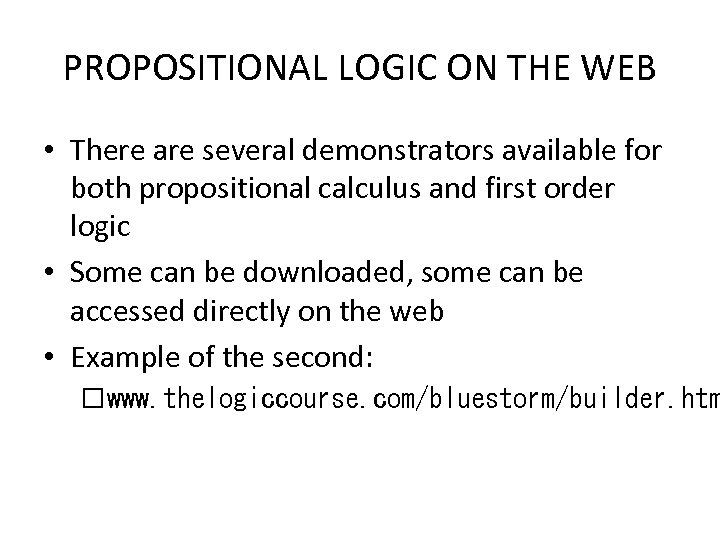PROPOSITIONAL LOGIC ON THE WEB • There are several demonstrators available for both propositional calculus and first order logic • Some can be downloaded, some can be accessed directly on the web • Example of the second: www. thelogiccourse. com/bluestorm/builder. htm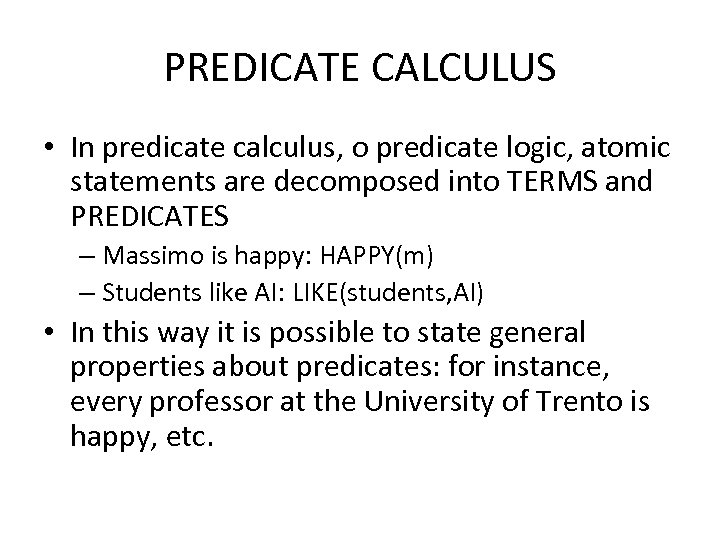PREDICATE CALCULUS • In predicate calculus, o predicate logic, atomic statements are decomposed into TERMS and PREDICATES – Massimo is happy: HAPPY(m) – Students like AI: LIKE(students, AI) • In this way it is possible to state general properties about predicates: for instance, every professor at the University of Trento is happy, etc.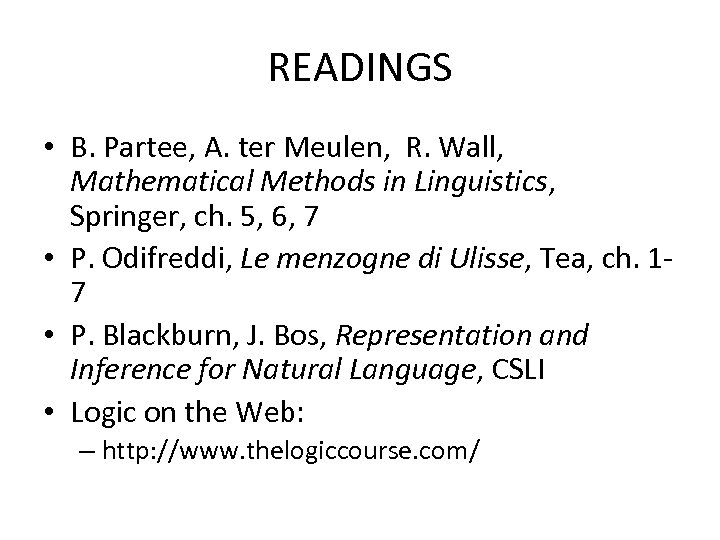READINGS • B. Partee, A. ter Meulen, R. Wall, Mathematical Methods in Linguistics, Springer, ch. 5, 6, 7 • P. Odifreddi, Le menzogne di Ulisse, Tea, ch. 17 • P. Blackburn, J. Bos, Representation and Inference for Natural Language, CSLI • Logic on the Web: – http: //www. thelogiccourse. com/